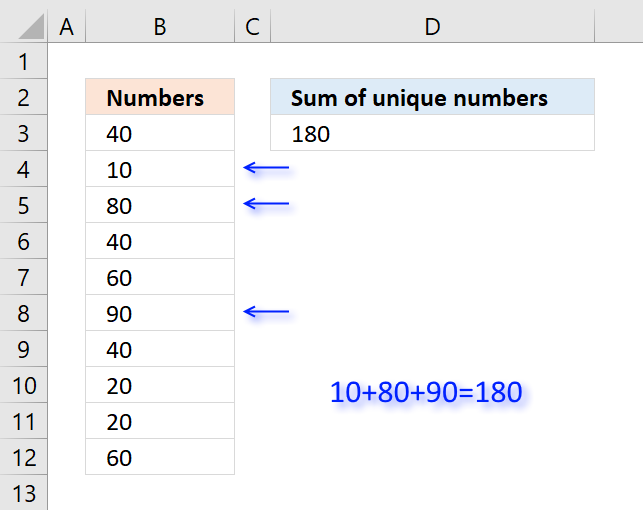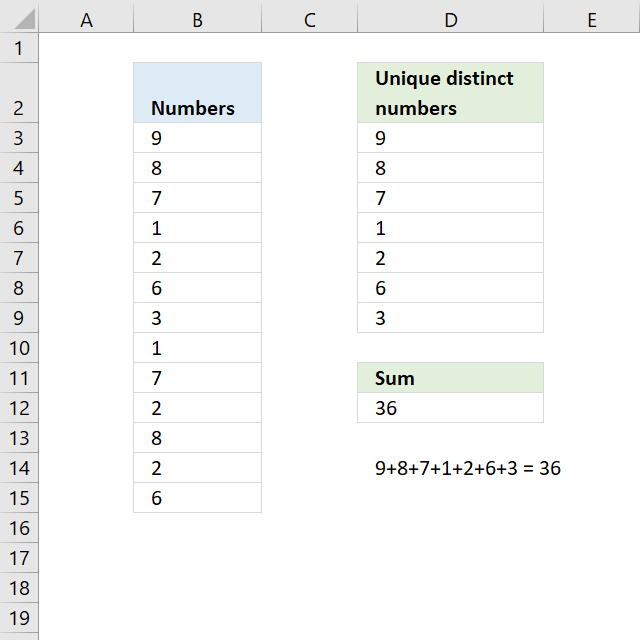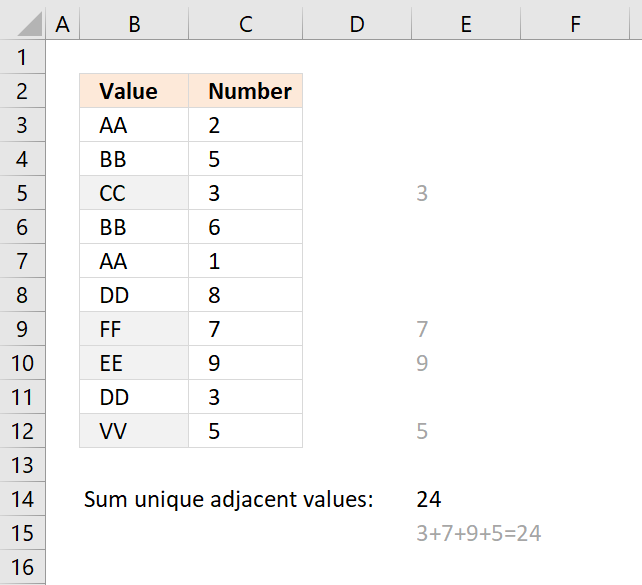Author: Oscar Cronquist Article last updated on March 16, 2022## 1. Sum unique numbersThe formula in cell D3 adds all unique numbers in cell range B3:B12 and returns the total. Unique values are all values occurring only once in the list.

Formula in cell D3:

=SUMPRODUCT((COUNTIF(B3:B12,B3:B12)=1)*B3:B12)

Excel 365 formula:

=SUM(UNIQUE(B3:B12,,TRUE))

### Explaining formula in cell D3

#### Step 1 - Count occurrence of each value in cell range

The COUNTIF function is an extremely useful function, this time I am counting how many times each value shows up in the cell range.

COUNTIF(B3:B12,B3:B12)

becomes

COUNTIF({40; 10; 80; 40; 60; 90; 40; 20; 20; 60},{40; 10; 80; 40; 60; 90; 40; 20; 20; 60})

and returns

{3;1;1;3;2;1;3;2;2;2}

#### Step 2 - Check if value is unique

The equal sign checks if number is equal to 1.

COUNTIF(B3:B12,B3:B12)=1

becomes

{3;1;1;3;2;1;3;2;2;2}=1

and returns

{FALSE; TRUE; TRUE; FALSE; FALSE; TRUE; FALSE; FALSE; FALSE; FALSE}

#### Step 3 - Multiply with cell range

The boolean values in the array show which numbers to include in sum. FALSE is the same as 0 (zero) and TRUE is equivalebt to 1. The parentheses make sure that the comparison is calculated before multiplying.

(COUNTIF(B3:B12,B3:B12)=1)*B3:B12

becomes

{FALSE; TRUE; TRUE; FALSE; FALSE; TRUE; FALSE; FALSE; FALSE; FALSE}*B3:B12

becomes

{FALSE; TRUE; TRUE; FALSE; FALSE; TRUE; FALSE; FALSE; FALSE; FALSE}*{3;1;1;3;2;1;3;2;2;2}

and returns

{0;10;80;0;0;90;0;0;0;0}

#### Step 4 - Sum numbers

The SUMPRODUCT function now simply adds the numbers and returns the total.

SUMPRODUCT((COUNTIF(B3:B12,B3:B12)=1)*B3:B12)

becomes

SUMPRODUCT({0;10;80;0;0;90;0;0;0;0})

and returns 180 in cell D3.

Why use the SUMPRODUCT function and not the SUM function? You don't need to enter this formula as an array formula if you use the SUMPRODUCT function.

### Get Excel *.xlsx file

Sum unique numbers.xlsx

## 2. Sum unique distinct numbersThe image above shows numbers in column B, some of these numbers are duplicates. The formula in D12 adds unique distinct numbers meaning the first instance of each number is added to the total, however, the duplicates are ignored.

=SUMPRODUCT((1/COUNTIF(B3:B15,B3:B15))*B3:B15)

Excel 365 dynamic array formula:

=SUM(UNIQUE(B3:B15, , FALSE))

### Explaining formula in cell D12

If you want to examine the formula yourself then go to tab "Formula" on the ribbon and then press with left mouse button on "Evaluate Formula" button.

This will show the calculation steps, one at a time.  Simply press with left mouse button on the Evaluate button to move to next calculation step.

#### Step 1 - Count each value in cell range

The COUNTIF function counts each value in the cell range and returns an array of numbers which shows the number of instances each value has.

COUNTIF(B3:B15,B3:B15)

becomes

COUNTIF({9;8;7;1;2;6;3;1;7;2;8;2;6},{9;8;7;1;2;6;3;1;7;2;8;2;6})

and returns

{1;2;2;2;3;2;1;2;2;3;2;3;2}.

#### Step 2 - Divide 1 with array

If a number has a duplicate then there are two instances of that value. Divide 1 with 2 and we get 0.5, now multiply that value with the number and we get the number to add to the total.

1/COUNTIF(B3:B15,B3:B15)

becomes

1/{1;2;2;2;3;2;1;2;2;3;2;3;2}

and returns

{1;0.5;0.5;0.5;0.333333333333333;0.5;1;0.5;0.5;0.333333333333333;0.5;0.333333333333333;0.5}

#### Step 3 - Multiply with numbers

The parentheses make sure that the order of calculation is correct.

(1/COUNTIF(B3:B15,B3:B15))*B3:B15

becomes

{1;0.5;0.5;0.5;0.333333333333333;0.5;1;0.5;0.5;0.333333333333333;0.5;0.333333333333333;0.5}*B3:B15

and returns

{9;4;3.5;0.5;0.666666666666667;3;3;0.5;3.5;0.666666666666667;4;0.666666666666667;3}

#### Step 4 - Add numbers

The SUMPRODUCT function is a better option than the SUM function, we don't need to enter the formula as an array formula now.

SUMPRODUCT((1/COUNTIF(B3:B15,B3:B15))*B3:B15)

becomes

SUMPRODUCT({9;4;3.5;0.5;0.666666666666667;3;3;0.5;3.5;0.666666666666667;4;0.666666666666667;3})

and returns 36 in cell D12.

### Get Excel *.xlsx file

Sum unique distinct numbers.xlsx

## 3. Sum number based on the corresponding unique valueThe formula in cell E14 adds a number from column C if the corresponding value in column B is unique and returns the total for all unique values. Unique values are values that exist only once in the list, in other words, there is only one instance of the value.

Formula in cell E14:

=SUMPRODUCT((COUNTIF(\$B\$3:\$B\$12,\$B\$3:\$B\$12)=1)*\$C\$3:\$C\$12)

### Explaining formula in cell E14

#### Step 1 - Count values in column B

The COUNTIF function counts values based on a condition, I am using multiple conditions, in this case.

COUNTIF(\$B\$3:\$B\$12,\$B\$3:\$B\$12)

becomes

COUNTIF({"AA";"BB";"CC";"BB";"AA";"DD";"FF";"EE";"DD";"VV"}, {"AA";"BB";"CC";"BB";"AA";"DD";"FF";"EE";"DD";"VV"})

and returns

{2;2;1;2;2;2;1;1;2;1}

#### Step 2 - Check if unique

The equal sign compares each value in the array to 1 and returns either TRUE or FALSE.

COUNTIF(\$B\$3:\$B\$12,\$B\$3:\$B\$12)=1

becomes

{2;2;1;2;2;2;1;1;2;1}=1

and returns

{FALSE; FALSE; TRUE; FALSE; FALSE; FALSE; TRUE; TRUE; FALSE; TRUE}

#### Step 3 - Multiply with numbers in column C

(COUNTIF(\$B\$3:\$B\$12,\$B\$3:\$B\$12)=1)*\$C\$3:\$C\$12

becomes

{FALSE; FALSE; TRUE; FALSE; FALSE; FALSE; TRUE; TRUE; FALSE; TRUE}*\$C\$3:\$C\$12

becomes

{FALSE; FALSE; TRUE; FALSE; FALSE; FALSE; TRUE; TRUE; FALSE; TRUE}*{2;5;3;6;1;8;7;9;3;5}

and returns

{0;0;3;0;0;0;7;9;0;5}

#### Step 4 - SUM numbers

The SUMPRODUCT function then adds the numbers and returns the total to cell E14.

SUMPRODUCT((COUNTIF(\$B\$3:\$B\$12,\$B\$3:\$B\$12)=1)*\$C\$3:\$C\$12)

becomes

SUMPRODUCT({0;0;3;0;0;0;7;9;0;5})

and returns

24 in cell E14.

### Get Excel *.xlsx file

Sum only if unique value in another column.xlsx